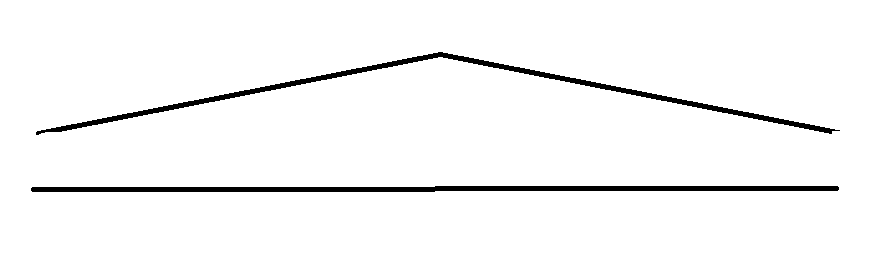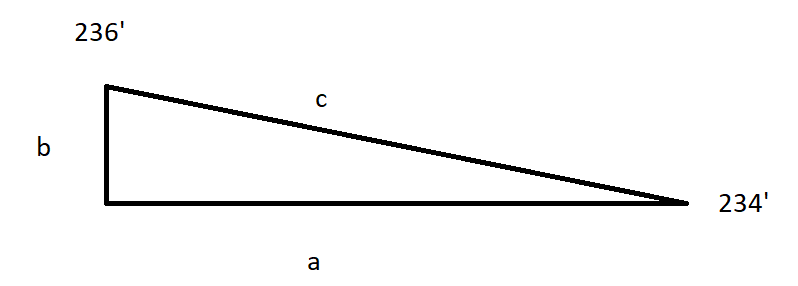# Calculate area lost due to expansion of roads in mountainous terrain

305
8
07-02-2019 10:54 AMNew Contributor

I am trying to find out the area of land that is lost due to the expansion of an existing road in mountainous terrain. Suppose, the road width needs to be increased from 40 ft to 80 ft, how do I go about calculating the area of land lost to this road. I am sure the additional 40 ft expansion doesn't mean 400 sq ft if the length of the road were to be 10 ft in mountainous terrain. Any leads on how I can calculate this?

8 RepliesFrequent Contributor III

L2 x W2 - L1 x W1Frequent Contributor III

If you want to be more precise buffer the initial center line by 20 (makes 40 foot wide when done)

Then create a second buffer using 40 feet.  the shape area field created will give you a precise set of numbers.

10000 feet of road at 40 feet = 400000 ft square.

10000 feet of road at 80 feet = 800000

Essentially you double the width of a road you double the area.New Contributor

Thanks for your reply! But the problem is I am looking at a mountainous terrain and not a flat terrain. So the surface area lost would be slightly higher than if the road were built on a flat surface? Any pointers to calculate this using ArcGIS?Frequent Contributor III

do you have 3D Analyst?

If not there is not much to tell you.

If you do then there is way to much to tell you about how to get it done the easy way

The diagram below simplifies your issue. This represents the same stretch of road. from the side the elevation changes. Hence the road on top is longer in reality.here is a work around.  If you only have 10 miles or so not an issue.

working on a copy of your data

get the contours for your area.  even intervals  i.e. 2 feet, 1 foot etc.

if your contours are 2 foot.  use simple math in an excel spreadsheeta =  shape length

b =  absolute change in elevation

c =  calculated length

In reality this should work on hundreds of miles of road as easily as it would on a mile.  Just a larger spreadsheet.Frequent Contributor III

So if your segment is 100 feet long in the shape length the actual length would be

100.019998 your change in area would be 4,000.79992

add them all up and you get a pretty close estimation of change in area.New Contributor

Thank you again! Yes, I do have 3D analyst. I understood the point till creating contours. But, the rest of the calculations are to be done in a spreadsheet? Is there a specific example I can look at for reference?Frequent Contributor IIII took out the =  sign so the formula would show.  since the area would double you only need to calculate 40 footFrequent Contributor III

What I meant was get the contours.  In my state we have lidar derived contours.

We had a similar project.  I acquired the lidar data and created 1 foot contours.  I think there are tools with analyst to calculate what your looking for based on slope.  Ours was a little more tricky in that it was for transmission lines and we tried to calculate in also the sag in the line between towers.